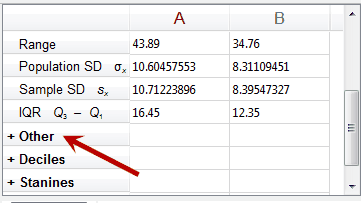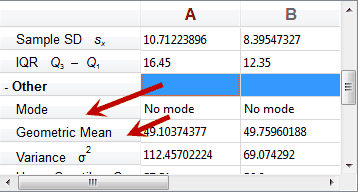﻿ Expanding Subsections

# Expanding Subsections

Top  Previous  Next

Each statistics column contains a large number of statistics.  One variable statistics are broken into five sections.

 • Main  -  Mean, Median, Minimum, Maximum, Range, Standard Deviation, Sample standard deviation and Inter-quartile range
 • Other -  Mode, Geometric Mean, Variance, Upper Quartile, Lower Quartile, Mean Absolute Deviation, Sum and Sum of squares
 • Deciles
 • Stanines
 • Percentiles

Two variable statistics are also broken into sections.

 • Regression Information (which is dependent on the current regression model selected).
 • Summary Statistics for First Column
 • Summary Statistics for Second Column.

You can expand or contract each section by clicking on the section header.